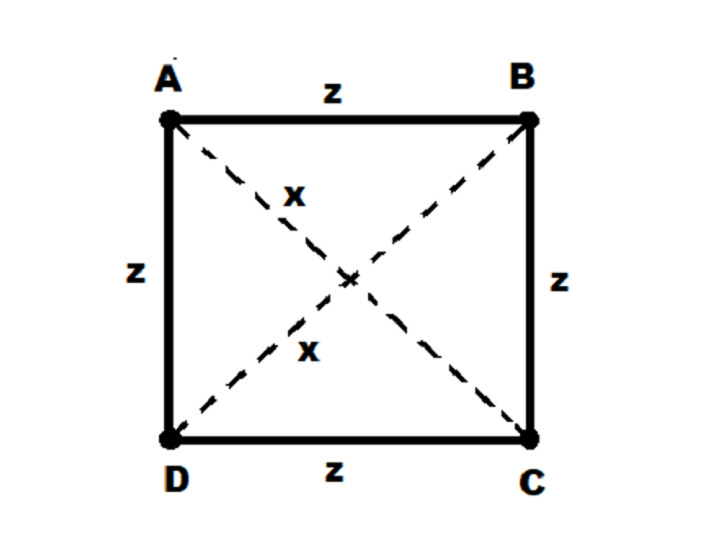# How to Confirm If Given Four Points Form a Square in Java?

A square is a two-dimensional shape which has four sides of equal length. The opposite sides of a square are parallel to each other and all four interior angles are right angles with diagonal of equal length. In this article we will check how to confirm if given four points form a square.

We will be given a square with four points i.e. A, B, C, D as shown in the figure −We need to check from these points that if they form a square or not. To check this it should satisfy the following condition −

• The distance between the points A and C, and the points B and D i.e. “x” should be equal.

• The distance between the points A and B, and the points B and C, and the points C and D, and the points D and A i.e. “z” should be equal.

We will find the distance between two points using the formula −

$$\mathrm{d=\sqrt{(x_{2}-x_{1})^2(y_{2}-y_{1})^2}}$$

where the point 1 will be (x1, y1) and point 2 will be (x2, y2).

Let’s start!

## To show you some instances

### Instance-1

• Given four inputs points are −

• P1(3,7), P2(4,3), P3(7,8), P4(1,9)

• After putting it in distance formula and checking condition for square the result will be −

• Given four points do not form a square.

### Instance-2

• Given four inputs points are −

• P1(20,20), P2(20,10), P3(10,10), P4(10,20)

• After putting it in distance formula and checking condition for square the result will be −

• Given four points form a square.

### Algorithm

• Step-1 − Declare and initialize the variables.

• Step-2 − Find the distance between center 1 and center 2 of the circle.

• Step-3 − Check for the five conditions of distance.

• Step-4 − Print the result.

## Multiple Approaches

We have provided the solution in different approaches.

• By Using Static Inputs

• By Using User Defined Method

Let’s see the program along with its output one by one.

## Approach-1: By Using Static Input

In this approach, Points value will be assigned. Then as per the algorithm we will find if given four points form a square.

### Example

public class Main{

//main method
public static void main(String[] args){

//declaring variables
int x1=3, x2=4, x3=7, x4=1;
int y1=7, y2=3, y3=8, y4=9;
double d1, d2, d3, d4, d5, d6;

//applyinng logic
d1 = (x2 - x1) * (x2 - x1) + (y2 - y1) * (y2 - y1);
d2 = (x3 - x2) * (x3 - x2) + (y3 - y2) * (y3 - y2);
d3 = (x4 - x3) * (x4 - x3) + (y4 - y3) * (y4 - y3);
d4 = (x1 - x4) * (x1 - x4) + (y1 - y4) * (y1 - y4);
d5 = (x4 - x2) * (x4 - x2) + (y4 - y2) * (y4 - y2);
d6 = (x3 - x1) * (x3 - x1) + (y3 - y1) * (y3 - y1);

if (d1 == 0 || d2 == 0 || d3 == 0 || d4 == 0 || d5 == 0 || d6 == 0){
System.out.println("Given four points do not form a square");
}
else if (d1 == d2 && d2 == d3 && d3 == d4 && d5 == d6){

//prints if four points form square
System.out.println("Given four points form a square");
} else {

//prints if four points do not form square
System.out.println("Given four points do not form a square");
}

}
}


### Output

Given four points do not form a square


## Approach-2: By Using User Defined Method

In this approach, Points value will be assigned. Then call a user defined method by passing the given values and as per the algorithm we will find if given four points form a square.

### Example

public class Main{

//main method
public static void main(String[] args){

//creating objects of Point
Point p1 = new Point(20, 20);
Point p2 = new Point( 20, 10 );
Point p3 = new Point(10, 10 );
Point p4 = new Point( 10, 20 );

//calling user defined method
if(isSquare(p1, p2, p3, p4)==true){

//print if four points form a square
System.out.println("Given four points form a square");
}
else{

//print if points does not form a square
System.out.println("Given four points do not form a square");
}
}

// Declaring Point class
static class Point{
int x, y;
public Point(int x, int y){
this.x = x;
this.y = y;
}
};

//function to find square of distance from point 'p' to point 'q'
static int distSq(Point p, Point q){
return (p.x - q.x) * (p.x - q.x) + (p.y - q.y) * (p.y - q.y);
}

//user defined method
static boolean isSquare(Point p1, Point p2, Point p3, Point p4){
int d1 = distSq(p1, p2);
int d2 = distSq(p2, p3);
int d3 = distSq(p3, p4);
int d4 = distSq(p4, p1);

int d5 = distSq(p1, p3);
int d6 = distSq(p2, p4);
if (d1 == 0 || d2 == 0 || d3 == 0 || d4 == 0 || d5 == 0 || d6 == 0)
return false;

if (d1 == d2 && d2 == d3 && d3 == d4 && d5 == d6){

//it returns true if (p1, p2, p3, p4) form a square
return true;
}

//it returns false if (p1, p2, p3, p4) do not form a square
return false;
}
}


### Output

Given four points form a square


In this article, we explored different approaches to check if a line touches, intersects or lies outside a circle by using Java programming language.

Updated on: 04-May-2023

216 Views